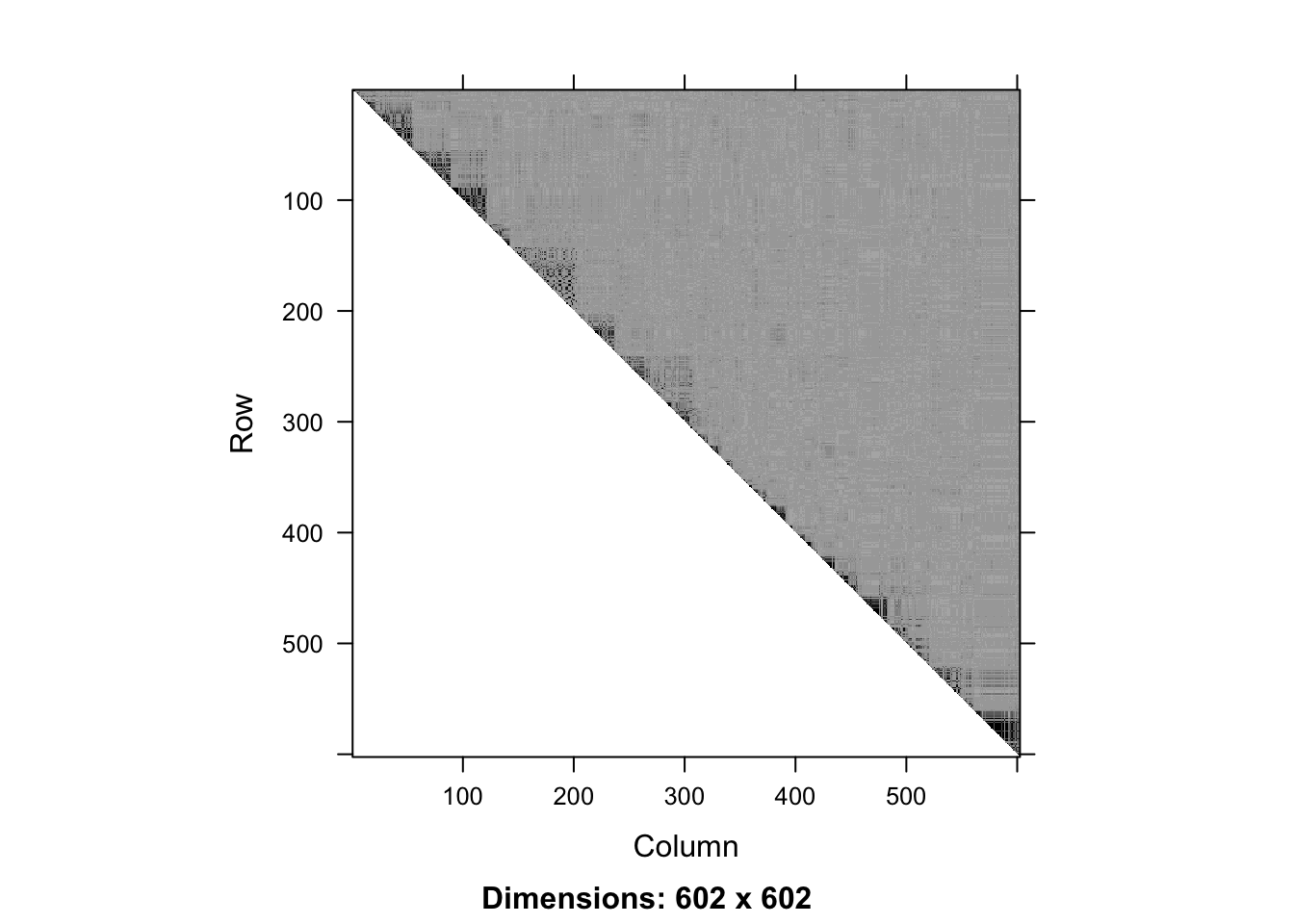# this vignette is not created if snpStats is not installed
if (!require("snpStats")) {
knitr::opts_chunk\$set(eval = FALSE)
}
##
## Attaching package: 'Matrix'
## The following object is masked from 'package:S4Vectors':
##
##     expand

Introduction

In this vignette we demonstrate the use of snpClust function in the adjclust package. snpClust performs adjacency-constrained hierarchical clustering of single nucleotide polymorphisms (SNPs), where the similarity between SNPs is defined by linkage disequilibrium (LD).

This function implements the algorithm described in the third chapter of . It is an extension of the algorithm described in . Denoting by \(p\) the number of SNPs to cluster and assuming that the similarity between SNPs whose indices are more distant than \(h\), its time complexity is \(O(p (\log(p) + h))\), and its space complexity is \(O(hp)\).

The beginning of this vignette closely follows the "LD vignette" of the SnpStats package . First, we load genotype data.

data("ld.example", package = "snpStats")

We focus on the ceph.1mb data.

geno <- ceph.1mb[, -316]  ## drop one SNP leading to one missing LD value
p <- ncol(geno)
nSamples <- nrow(geno)
geno
## A SnpMatrix with  90 rows and  602 columns
## Row names:  NA06985 ... NA12892
## Col names:  rs5993821 ... rs5747302

These data are drawn from the International HapMap Project and concern 602 SNPs1 over a 1Mb region of chromosome 22 in sample of 90 Europeans.

We can compute and display the LD between these SNPs.

ld.ceph <- snpStats::ld(geno, stats = "R.squared", depth = p-1)
image(ld.ceph, lwd = 0)The snpClust function can handle genotype data as an input:

fit <- snpClust(geno, stats = "R.squared")
## Warning in run.snpClust(x, h = h, stats = stats): Forcing the LD similarity to
## be smaller than or equal to 1
## Note: 135 merges with non increasing heights.

Note that due to numerical errors in the LD estimation, some of the estimated LD values may be slightly larger than 1. These values are rounded to 1 internally.

The above figure suggests that the LD signal is concentrated close to the diagonal. We can focus on a diagonal band with the bandwidth parameter h:

fitH <- snpClust(geno, h = 100, stats = "R.squared")
## Warning in run.snpClust(x, h = h, stats = stats): Forcing the LD similarity to
## be smaller than or equal to 1
## Note: 133 merges with non increasing heights.
fitH
##
## Call:
## run.adjclust(mat = mat, type = type, h = h, strictCheck = strictCheck)
##
## Cluster method   : snpClust
## Number of objects: 602

Output

The output of the snpClust is of class chac. In particular, it can be plotted as a dendrogram silently relying on the function plot.dendrogram:

plot(fitH, type = "rectangle", leaflab = "perpendicular")
## Warning in plot.chac(fitH, type = "rectangle", leaflab = "perpendicular"):
## Detected reversals in dendrogram: mode = 'corrected', 'within-disp' or 'total-disp' might be more relevant.